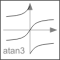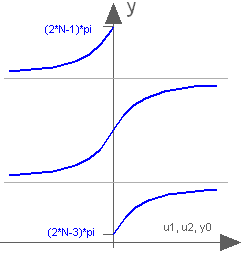# atan3

Four quadrant inverse tangent (select solution that is closest to given angle y0)# Information

This information is part of the Modelica Standard Library maintained by the Modelica Association.

This function returns y = atan3(u1,u2,y0) such that tan(y) = u1/u2 and y is in the range: -pi < y-y0 ≤ pi.
u2 may be zero, provided u1 is not zero. The difference to Modelica.Math.atan2(..) is the optional third argument y0 that allows to specify which of the infinite many solutions shall be returned:Note, for the default case (y0=0), exactly the same result as with atan2(..) is returned.

# Syntax

y = atan3(u1, u2, y0)

# Inputs (3)

u1 Type: Real Type: Real Default Value: 0 Type: Angle (rad) Description: y shall be in the range: -pi < y-y0 <= pi